Test: Networks Theorem

# Test: Networks Theorem

Test Description

## 20 Questions MCQ Test GATE Electrical Engineering (EE) 2023 Mock Test Series | Test: Networks Theorem

Test: Networks Theorem for Railways 2023 is part of GATE Electrical Engineering (EE) 2023 Mock Test Series preparation. The Test: Networks Theorem questions and answers have been prepared according to the Railways exam syllabus.The Test: Networks Theorem MCQs are made for Railways 2023 Exam. Find important definitions, questions, notes, meanings, examples, exercises, MCQs and online tests for Test: Networks Theorem below.
Solutions of Test: Networks Theorem questions in English are available as part of our GATE Electrical Engineering (EE) 2023 Mock Test Series for Railways & Test: Networks Theorem solutions in Hindi for GATE Electrical Engineering (EE) 2023 Mock Test Series course. Download more important topics, notes, lectures and mock test series for Railways Exam by signing up for free. Attempt Test: Networks Theorem | 20 questions in 60 minutes | Mock test for Railways preparation | Free important questions MCQ to study GATE Electrical Engineering (EE) 2023 Mock Test Series for Railways Exam | Download free PDF with solutions
 1 Crore+ students have signed up on EduRev. Have you?
Test: Networks Theorem - Question 1

### vTH , RTH = ?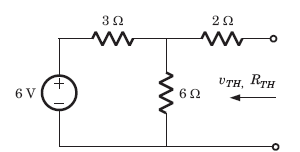Detailed Solution for Test: Networks Theorem - Question 1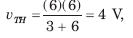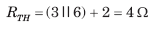Test: Networks Theorem - Question 2

### IN  , RN = ?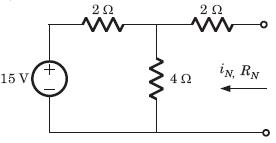Detailed Solution for Test: Networks Theorem - Question 2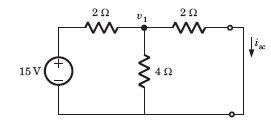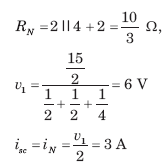Test: Networks Theorem - Question 3

### Which theorem assists in replacement of an impedance branch over the network by the other network comprising different circuit components, without affecting the V-I relations throughout the entire network?

Test: Networks Theorem - Question 4

A simple equivalent circuit of the 2 terminal network shown in fig.  is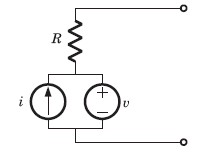Detailed Solution for Test: Networks Theorem - Question 4

After killing all source equivalent resistance is R
Open circuit voltage = v1

Test: Networks Theorem - Question 5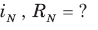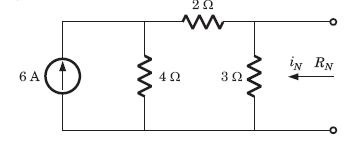Detailed Solution for Test: Networks Theorem - Question 5

The short circuit current across the terminal is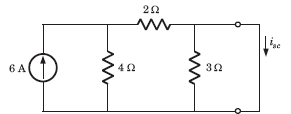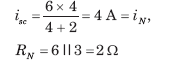Test: Networks Theorem - Question 6

VTH, RTH  = ?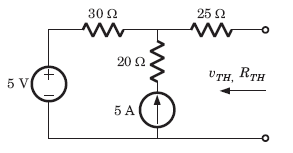Detailed Solution for Test: Networks Theorem - Question 6

For the calculation of RTH if we kill the sources
then 20Ω resistance is inactive because 5 A source will
be open circuit
RTH = 30+25 = 55 Ω,
vTH = 5+5X30 = 155V

Test: Networks Theorem - Question 7

RTH = ?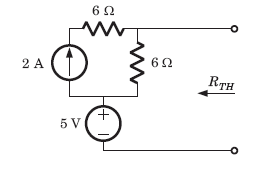Detailed Solution for Test: Networks Theorem - Question 7

After killing the source, RTH = 6 Ω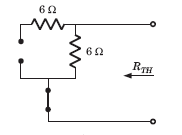Test: Networks Theorem - Question 8

The Thevenin impedance across the terminals ab of the network shown in fig. is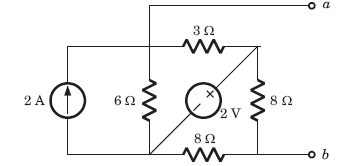Detailed Solution for Test: Networks Theorem - Question 8

After killing all source,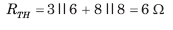Test: Networks Theorem - Question 9

For In the the circuit shown in fig. a network and its Thevenin and Norton equivalent are given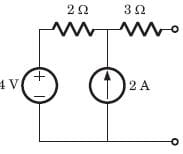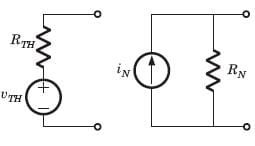The value of the parameter are

VTH  RTH  I RRN

Detailed Solution for Test: Networks Theorem - Question 9

v∝ = 2 x 2 + 4 = 8 V = vTH
RTH = 2 + 3 = 5Ω = RN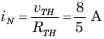Test: Networks Theorem - Question 10

v1 = ?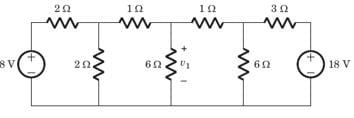Detailed Solution for Test: Networks Theorem - Question 10

If we solve this circuit direct, we have to deal with three variable. But by simple manipulation variable can be reduced to one. By changing the LHS and RHS in Thevenin equivalent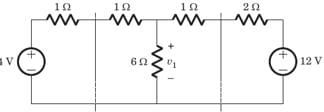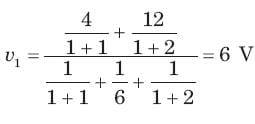Test: Networks Theorem - Question 11

i1 =  ?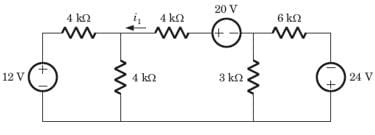Detailed Solution for Test: Networks Theorem - Question 11

If we solve this circuit direct, we have to deal with three variable. But by simple manipulation variable can be reduced to one. By changing the LHS and RHS in Thevenin equivalent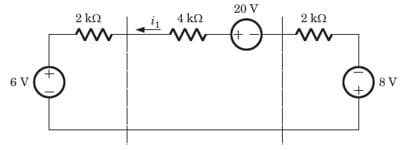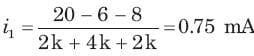Test: Networks Theorem - Question 12

A circuit is given in fig.  Find the Thevenin equivalent as given in question..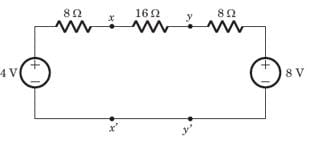As viewed from terminal x and x' is

Detailed Solution for Test: Networks Theorem - Question 12

We Thevenized the left side of xx'and source transformed right side of yy'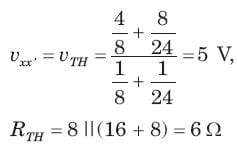Test: Networks Theorem - Question 13

A circuit is given in fig. Find theThevenin equivalent as given in question.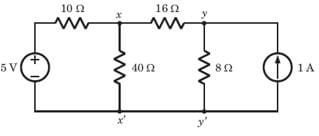As viewed from terminal y and y' is

Detailed Solution for Test: Networks Theorem - Question 13

Thevenin equivalent seen from terminal yy' is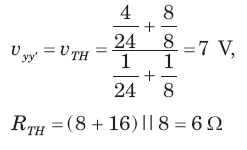Test: Networks Theorem - Question 14

A practical DC current source provide 20 kW to a 50Ω load and 20 kW to a 200 Ω load. The maximum power, that can drawn from it, is

Detailed Solution for Test: Networks Theorem - Question 14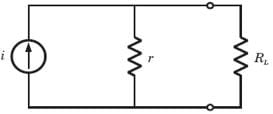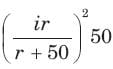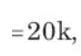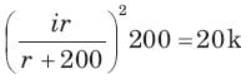(r + 200)2 = 4(r + 50)2
⇒ r = 100 Ω
i = 30A,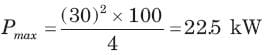Test: Networks Theorem - Question 15

In the circuit of fig. P.1.4.15–16 when R = 0 Ω , the current iR equals 10 A.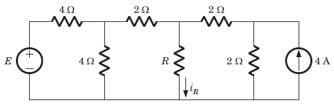The value of R, for which it absorbs maximum power, is

Detailed Solution for Test: Networks Theorem - Question 15

Thevenized the circuit across R,RTH = 2 Ω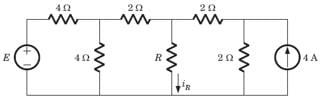Test: Networks Theorem - Question 16

In the circuit of fig when R = 0 Ω , the current iR equals 10 A.The maximum power will be

Detailed Solution for Test: Networks Theorem - Question 16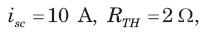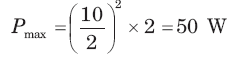Test: Networks Theorem - Question 17

A battery has a short-circuit current of 30 A and an open circuit voltage of 24 V. If the battery is connected to an electric bulb of resistance 2Ω, the power dissipated by the bulb is

Detailed Solution for Test: Networks Theorem - Question 17

rbattery VocIsc = 24/30 = 0.8 ohms

P = V2oc / ( r + 2)2   * 2

= 24/ ( .8 + 2)2  * 2

= 146.9 W

Test: Networks Theorem - Question 18

The following results were obtained from measurements taken between the two terminal of a resistive network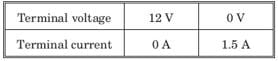The Thevenin resistance of the network is

Detailed Solution for Test: Networks Theorem - Question 18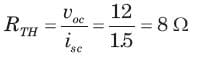Test: Networks Theorem - Question 19

A DC voltmeter with a sensitivity of 20 kΩ/V is used to find the Thevenin equivalent of a linear network. Reading on two scales are as follows

(a) 0 - 10 V scale  : 4 V
(b) 0 - 50 V scale : 5 V

The Thevenin voltage and the Thevenin resistance of the network is

Detailed Solution for Test: Networks Theorem - Question 19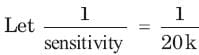= 50 μ A

For 0 - 10 V scale Rm = 10 x 20k = 200 kΩ
For 0 - 50 V scale Rm = 50 x 20k = 1 MΩ
For 4 V reading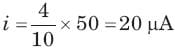vTH = 20μRTH + 20µ x 200k = 4 + 20μRTH     ...(i)
For 5V reading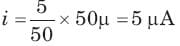UTH = 5μ x RTH + 5μ x 1M = 5 + 5μRTH        ...(ii)
Solving (i) and (ii)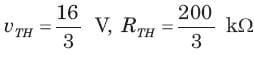Test: Networks Theorem - Question 20

Consider the network shown in fig.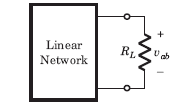The power absorbed by load resistance RL is shown in table :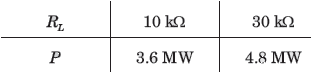Find the Thevenin equivalent.

Detailed Solution for Test: Networks Theorem - Question 20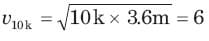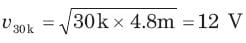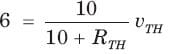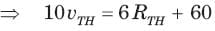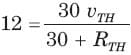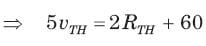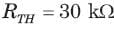## GATE Electrical Engineering (EE) 2023 Mock Test Series

22 docs|274 tests
 Use Code STAYHOME200 and get INR 200 additional OFF Use Coupon Code
Information about Test: Networks Theorem Page
In this test you can find the Exam questions for Test: Networks Theorem solved & explained in the simplest way possible. Besides giving Questions and answers for Test: Networks Theorem, EduRev gives you an ample number of Online tests for practice

## GATE Electrical Engineering (EE) 2023 Mock Test Series

22 docs|274 tests